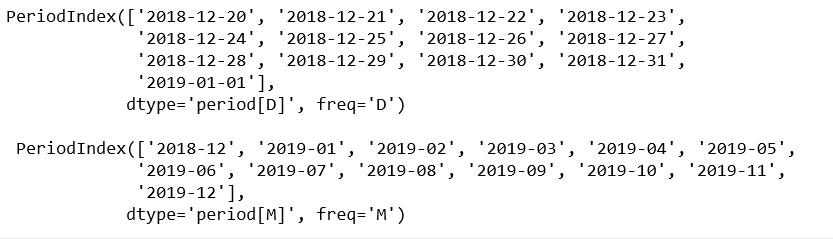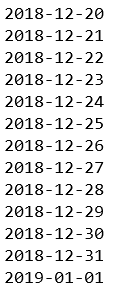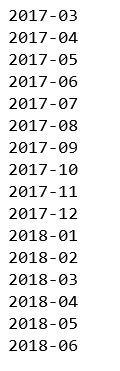# Python | pandas.period_range() method

Python is a great language for doing data analysis, primarily because of the fantastic ecosystem of data-centric python packages. Pandas is one of those packages and makes importing and analyzing data much easier.

`pandas.period_range()` is one of the general functions in Pandas which is used to return a fixed frequency PeriodIndex, with day (calendar) as the default frequency.

Syntax: pandas.to_numeric(arg, errors=’raise’, downcast=None)

Parameters:
start : Left bound for generating periods
end : Right bound for generating periods
periods : Number of periods to generate
freq : Frequency alias
name : Name of the resulting PeriodIndex

Returns: PeriodIndex

Code #1:

 `# importing pandas as pd ` `import` `pandas as pd ` ` `  `# period_range with freq = day ` `per1 ``=` `pd.period_range(start ``=``'2018-12-20'``, ` `              ``end ``=``'2019-01-01'``, freq ``=``'D'``) ` ` `  `# period_range with freq = month ` `per2 ``=` `pd.period_range(start ``=``'2018-12-20'``, ` `              ``end ``=``'2019-12-01'``, freq ``=``'M'``) ` ` `  `print``(per1, ``"\n\n"``, per2) `

Output:Code #2:

 `# importing pandas as pd ` `import` `pandas as pd ` ` `  `# period_range with freq = day ` `per1 ``=` `pd.period_range(start ``=``'2018-12-20'``,  ` `              ``end ``=``'2019-01-01'``, freq ``=``'D'``) ` ` `  `for` `val ``in` `per1: ` `    ``print``(val) `

Output:Code #3:

 `# importing pandas as pd ` `import` `pandas as pd ` ` `  `# Calling with pd.Period ` `per ``=` `pd.period_range(start ``=` `pd.Period(``'2017Q1'``, freq ``=``'Q'``), ` `                ``end ``=` `pd.Period(``'2018Q2'``, freq ``=``'Q'``), freq ``=``'M'``) ` ` `  `for` `val ``in` `per: ` `    ``print``(val) `

Output:My Personal Notes arrow_drop_upCheck out this Author's contributed articles.

If you like GeeksforGeeks and would like to contribute, you can also write an article using contribute.geeksforgeeks.org or mail your article to contribute@geeksforgeeks.org. See your article appearing on the GeeksforGeeks main page and help other Geeks.

Please Improve this article if you find anything incorrect by clicking on the "Improve Article" button below.

Article Tags :

Be the First to upvote.

Please write to us at contribute@geeksforgeeks.org to report any issue with the above content.